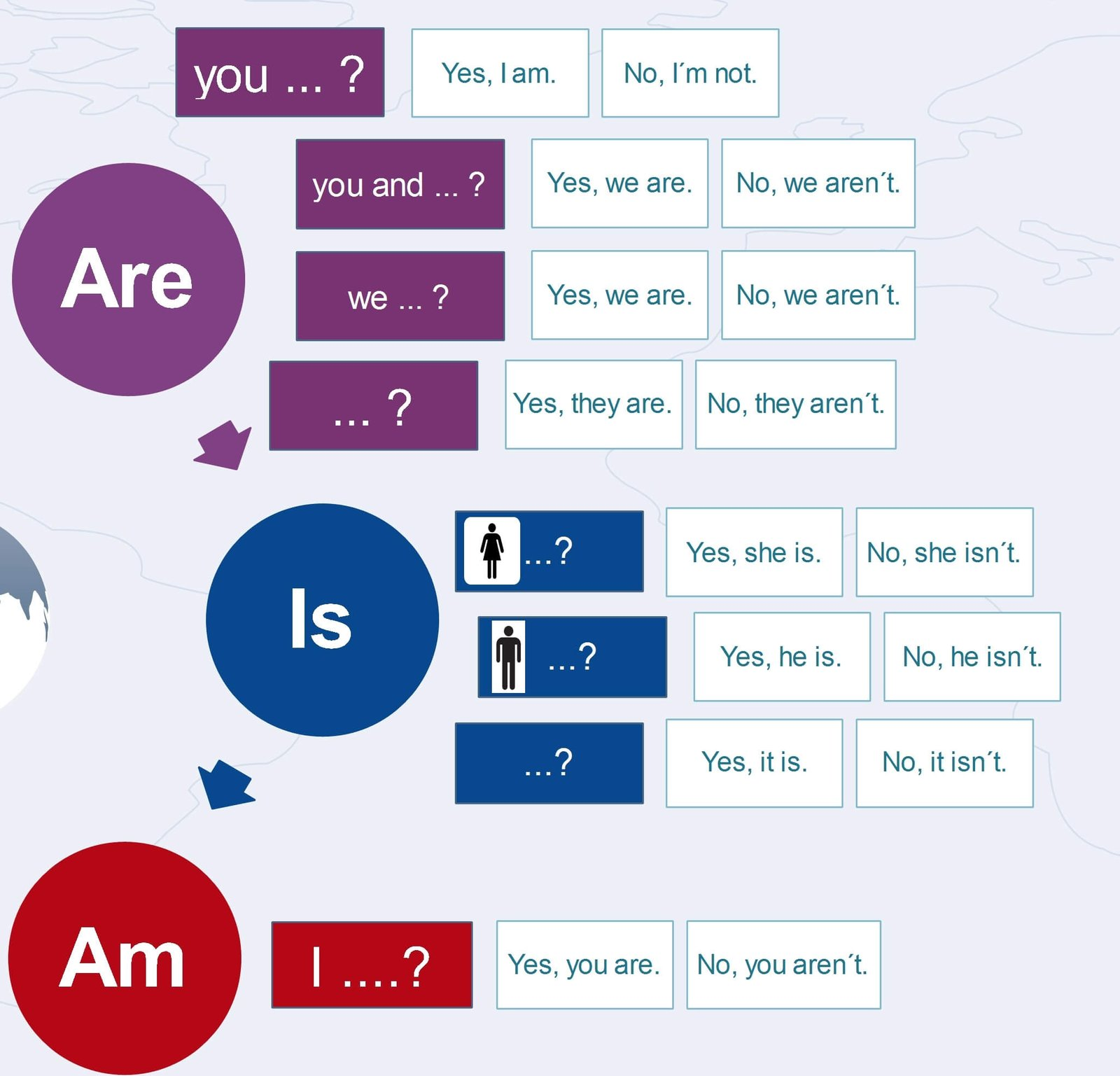# Present continuous questionsPresent continuous tense is one of the most popular tenses among students. It is easy to form and its usage is crystal clear (at least at the beginning). However, when it comes to forming questions, students often fail. To avoid this, I have come up with a number of activities to help my learners and I would like to share them with you now.
[showmyads] In this post, there is a video to teach the question word, an infographic, several interactive exercises and logical worksheets.

## Present continuous YES/NO questions

In the first part, it is important to teach the formation of the YES/NO questions in the present continuous tense. Print the following worksheet:
In the first part, there is a column of sentences which students should transform into questions. Tell them to use the colours to guide them. Help those struggling. Check the questions. Then tell students to transform the sentences in the second column.
Now your students should be able to form questions in the present continuous tense. And as they discovered the rules themselves, they are sure to remember them well.

## Present continuous tense – short answers

Display the following infographic.Demonstrate how the infographic works. Write one of the questions on the board. Ask the students with which word the question starts. Point to the word in the infographic. Elicit the colour. Now, point to the question on the board and elicit what follows the first word. Find the continuation in the rectangles and explain that the correct answers are the two possibilities behind it.
It took just two examples for my students to understand the formation of the short answers.

## Question words – video and infographic

Before you start teaching questions with the question words, teach the question words first. Here is a video teaching the question words:

You can use the following infographic too:Once the students know the basic question words, it is time to practise them. In the following video students see the answer and their task is to supply the correct question word.

## Present continuous questions

Now teach students how to form questions starting with WH… words in the present continuous tense.
Print the following worksheet.

Check the answers and tell them to complete the second column.

Finish the worksheet above in one lesson and then bring the following worksheet. This worksheet contains three exercises. In the first exercise students see the answer and their task is to write the question words. In the second task, students see the answer and they should ask about the underlined information. In the last exercise, students see the answer and they should write three questions for the given answer.

## Present continuous questions – more exercises

In the first quiz, you should form the YES/NO questions.
In the second quiz students have to write the short answers:
In the third quiz students know the answers and they have to write the questions.
In the fourth quiz, students have some cues and they have to write the questions using the cues.
If you cannot use a computer in your classroom, you can use the following worksheet with all the exercises.
(Visited 1,054 times, 1 visits today)1.Taha tadmori says: